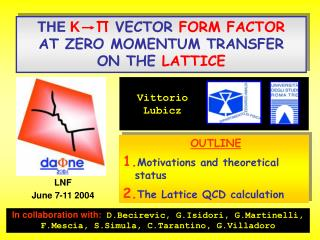Download PresentationOUTLINE Motivations and theoretical status The Lattice QCD calculationOUTLINE Motivations and theoretical status The Lattice QCD calculation - PowerPoint PPT Presentation

Download PresentationOUTLINE Motivations and theoretical status The Lattice QCD calculation
An Image/Link below is provided (as is) to download presentation

Download Policy: Content on the Website is provided to you AS IS for your information and personal use and may not be sold / licensed / shared on other websites without getting consent from its author. While downloading, if for some reason you are not able to download a presentation, the publisher may have deleted the file from their server.

- - - - - - - - - - - - - - - - - - - - - - - - - - - E N D - - - - - - - - - - - - - - - - - - - - - - - - - - -
Presentation Transcript

1. THE K ΠVECTOR FORM FACTOR AT ZERO MOMENTUM TRANSFER ON THE LATTICE Vittorio Lubicz OUTLINE Motivations and theoretical status The Lattice QCD calculation LNF June 7-11 2004 In collaboration with: D.Becirevic, G.Isidori, G.Martinelli, F.Mescia, S.Simula, C.Tarantino, G.Villadoro

2. 1) Vus=λis a fundamental SM parameter 2) Vud, Vus provide the most stringent test of unitarity “OLD” (2002) PDG Averages |Vud| = 0.9734 ± 0.0008 Superallowed and neutron β-decay |Vus| = 0.2196 ± 0.0026 K→πeν 3) Vus=λ and Vcb=Aλ2are crucial inputs in the Unitarity Triangle Analysis |Vub| = 0.0036 ± 0.0007 b→u inclusive and exclusive |Vud|2 + |Vus|2 + |Vub|2- 1 = -0.0042 ± 0.0019 MOTIVATIONS

3. The NEW experimental results BNL-E865 PRL 91, 261802 (Moriond ’04) KTeV hep-ex/0406001 KLOE Moriond ‘04

4. Vus = λ l ΓKl3= C SEW (1+δK) IKf+(0)2 2 5 GF|Vus|2 MK l l l v 192 π3 s u K π d ChPT: “Standard” estimate: Leutwyler, Roos (1984) (QUARK MODEL) f4 = −0.016 ± 0.008 f+(0) = 1 + f2 + f4 + O(p8) Vector Current Conservation f2 = − 0.023 Independent of Li (Ademollo-Gatto) THE LARGEST UNCERTAINTY Theoreticaldescription THE LARGEST UNCERTAINTY IS DUE TO THE FORM FACTOR AT ZERO MOMENTUM TRANSFER: f+(0)

5. f4 =Δloops(μ)− [C12 (μ) + C34 (μ)] (MK − Mπ) 8 2 2 2 ... and models LOC Jamin et al.,f4= -0.018 ± 0.009[Coupled channel dispersive analysis] LOC Cirigliano et al.,f4= -0.012[Resonance saturation] LOC Cirigliano et al.,f4= -0.016 ± 0.008[QM, Leutwyler and Roos] μ= ???Δloops(1GeV) = 0.004Δloops(Mρ) = 0.015Δloops(Mη) = 0.031 Fπ 4 Cirigliano et al.,f+ (0)= 0.981 ± 0.010 K0π- ChPT: The complete O(p6) calculation Post, Schilcher (2001), Bijnens, Talavera (2003) C12 (μ)andC34 (μ)can be determined from the slope and the curvature of the scalar form factor. Experimental data, however, are not accurate enough.

6. 1) Evaluation of f0(qMAX) with very high precision (>1%) 2 2) Extrapolation of f0(qMAX) to f0(0)=f+(0) by evaluating the slope λ0 2 The result is:Δf = - 0.017 ± 0.005stat ± 0.007syst(very close to LR!) + quenching error ! f+ (0) = 0.960 ± 0.005stat ± 0.007syst K0π- Lattice QCD calculation: the strategy D.Becirevic, G.Isidori, V.L., G.Martinelli, F.Mescia, S.Simula, C.Tarantino, G.Villadoro. hep-ph/0403217 3) Subtraction of the chiral logs (we compute Δf ≡ f+(0)-1-f2 ) and extrapolation to the physical meson masses

7. 1) Evaluation of f0(qMAX) 2 The basic ingredient is a double ratio of correlation functions: [FNAL]

8. 2) Extrapolation off0(qMAX) to f0(0) 2 Comparison of polar fits: LQCD:λ+=(25±2)10-3λ0=(12±2)10-3 KTeV:λ+=(24.11±0.36)10-3λ0=(13.62±0.73)10-3

9. The Ademollo-Gatto theorem f+(0)-1-f2 QUEN R= f(0) = 1+O[(MK − Mπ)] 2 2 2 (MK − Mπ) 2 2 2 Computed in Quenched-ChPT 3) Subtraction of the chiral logs and chiral extrapolation

10. Several functional forms are considered in the chiral extrapolation The final result is: Δf = - 0.017 ± 0.005stat ± 0.007syst [Leutwyler and Roos: ΔfLR = - 0.016 ± 0.008 ] f+ (0) = 0.960 ± 0.005stat ± 0.007syst = 0.960 ± 0.009 K0π- [Quenching error is not included] The dominant contributions to the systematic error come from the uncertainties on the q2 and mass dependencies of the form factor

11. CONCLUSIONS ♦We presented a quenched lattice study of the K→π vector form factor at zero momentum transfer ♦The calculation is the first one obtained by using a non-perturbative method based only on QCD, except for the quenched approximation ♦Our final result, f+ (0) = 0.960 ± 0.005stat ± 0.007systis in goodagreement with the estimate made by Leutwyler and Roos and quoted by the PDG ♦In order to increase the accuracy of the theoretical prediction the most important step is to remove the quenched approximation